Breaking News
Home / Land Surveying / Types Of Curves In Surveying Work

# Types Of Curves In Surveying Work

### What Is The Curve?

The Curves are generally the horizontal &/or vertical bends that are usually used on highways & the railways when it is necessary to change the alignment of the route. when 2 points are located at the diﬀerent levels, it becomes necessary to give the curve.

This curve usually helps to connect the points lying at the diﬀerent levels in such a way that the vehicles moving on that route could move with ease, safety & comfort. A proper alignment or the curve can provide smoother movement of the vehicles from 1 point to another, located at the diﬀerent levels.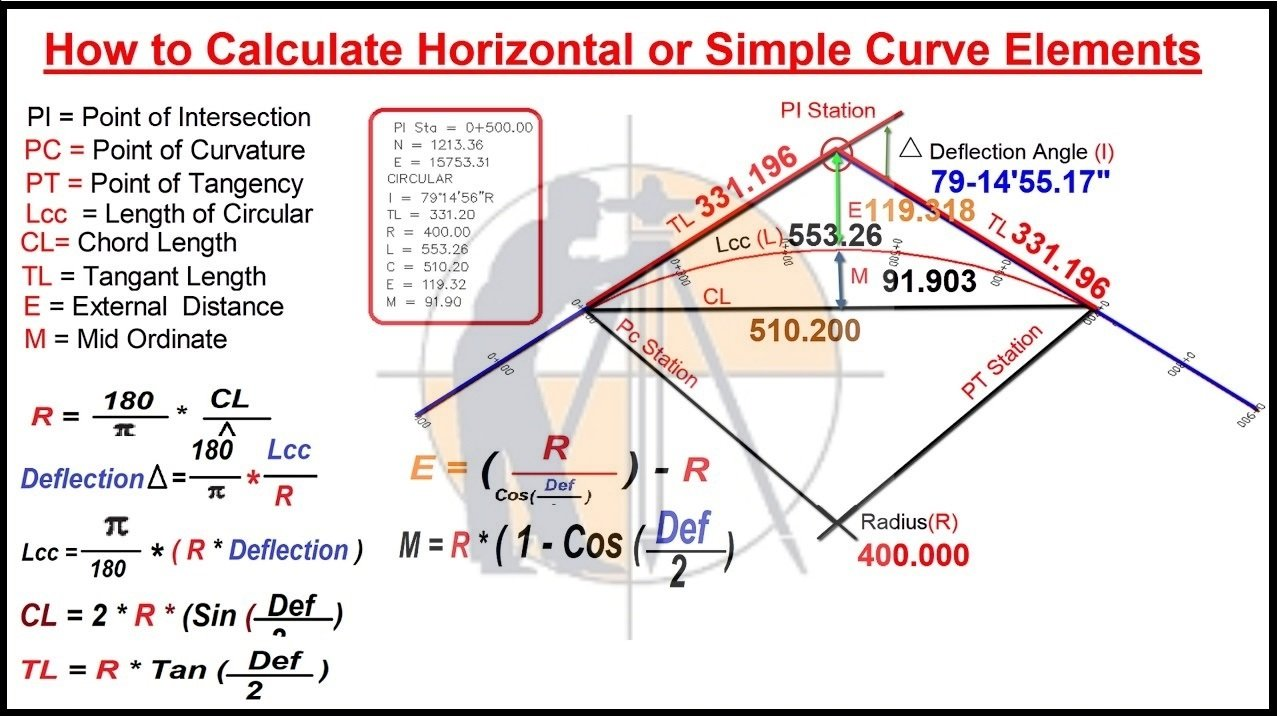A curve is introduced at the intersection of 2 straights to eﬀect the gradual change in the direction. This change in direction may be in the horizontal plane or a vertical plane. So curve is provided to the route according to its direction, i.e. either in the vertical plane or in a horizontal plane, respectively.

### Types Of Curves In Surveying Work

Curves in the surveying are classified into 2 main types. They are as follows.

1. Horizontal curves.
2. Vertical curves.

### 1 – Horizontal Curves.

The horizontal curve is provided where 2 straight lines intersect with each other in the horizontal plane. When a curve is given in the horizontal plane, it is known as a horizontal curve. A horizontal curves are further divided as follows:

1. Simple curve.
2. Compound curve.
3. Reverse curve.
4. Transition curve.
5. Combined curve.

### a) – Simple Curve.

The simple curve is a single arc of the circle, which is tangential to both the straight lines of route. There are a few elements of the simple circular curve discussed below.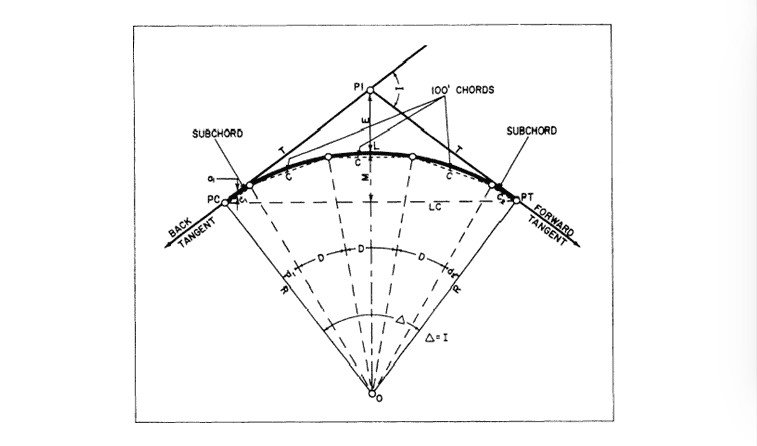### Back Tangent.

The line of the tangent before the beginning of the curve is known as a back tangent.

### Forward Tangent.

The tangent line after finishing of the curve is known as the forward tangent.

### Point Of the Intersection.

The point where the back tangent & the forward tangent intersects, is known as the point of intersection.

### Intersection Angle.

The angle between the back & the forward tangent is known as intersection angle.

### Angle Of Deflection.

An the angle through which the forward tangent deflects is known as angle of deflection.

### Point of curvature.

The point at the beginning of the curve, where the alignment changes the tangent into the curve, is known as point of the curvature.

### Point Of Tangency.

The point at the end of the curve, where the curve changes into the tangent, is known as point of the tangency.

### Tangent distance.

The distance between the point of the curvature & point of intersection is known as the tangent distance.

### Length Of Curve.

The total length of curve from the point of curvature to the point of intersection is known as the length of curve.

### Long Chord.

The chord joining the point of curvature & the point of tangency, is known as the long chord.

### Normal Chord.

The chord between 2 successive pegs on the curve, is known as a normal chord.

### Sub-chord.

The chord shorter than the normal chord is known as a sub-chord.

### Mid-ordinate.

The distance between the midpoint of the curve & the midpoint of the long chord, is known as mid-ordinate.

### External Distance.

The distance between the point of intersection & the midpoint of the curve, is the external distance.

### b) – Compound Curve.

The compound curve comprises 2 or more circular arcs of the diﬀerent radii with their centers of the curvature on the same side of the common tangent. It is where the cutting & filling of soil is to be avoided. Compound curves are necessary whenever the space restrictions rule out the signal circular curve & when there are property boundaries.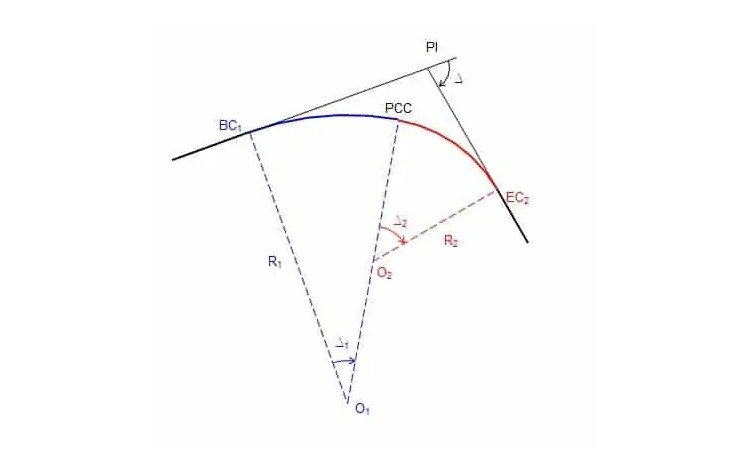### c) – Reverse Curve.

The curve consisting of 2 circular arcs of similar or diﬀerent sizes radii having their centers on opposite sides of the common tangent at the point of reverse curvature is known as a reverse curves reverse curve is also known as the serpentine curve or S-curve due to its shape. The Reverse curves are used to connect 2 parallel roads or railway lines. It is generally used when 2 lines intersect at a very small angle.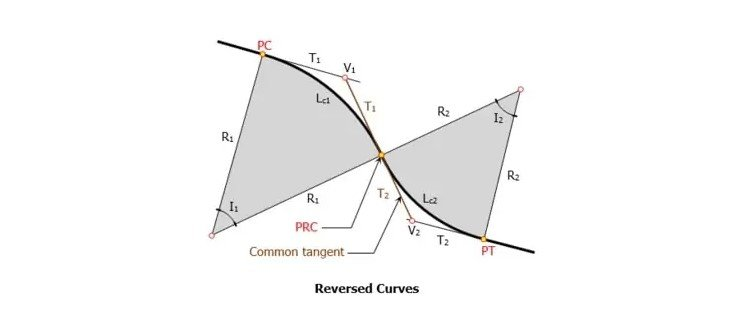The Reverse curves are best suited for hilly terrains & the highways used for relatively low-speed vehicles. Reverse curves are not advisable to use on the highways & railways which are meant for high-speed traﬃc movement because of the following reasons.

• ### What is the Benefits and Drawbacks of Total Station

1) – The sudden change in direction can be dangerous for the vehicles.

2) – The sudden change in the curvature & direction reduces the life of vehicles & also provides discomfort to the people traveling on that route.

3) – If the driver is the careless, it may cause the vehicle to overturn over the reverse curve.

### d) – Transition Curve.

It is the curve of varying radius. The value of the radius of this type of the curve varies from infinity to a certain fixed value. It provides the gradual change from the straight line to the circular curve & again from the circular curve to the straight line. It is usually provided on both ends of the circular curve. The transition curves are provided on the roads & railways to lessen the discomfort at the sudden change in the curvature at the junction of a straight line & a curve.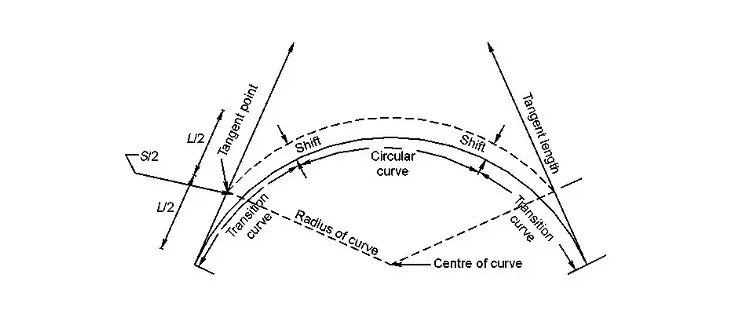The transition curve has the following advantages.

1) – Transition curve reduces the probability of the overturning vehicles at the junction of the straight and the curve.

2) – It gives comfort to the passengers.

3) – It allows higher speeds for the vehicles at the curves.

4) – Transition curve reduces the wear & tear of the rail section, occurring due to unusual friction at the point of curve.

### v) – Combined Curve

The combination of the simple circular curve & the transition curve, is known as a combined curve. Combined curves are mostly preferred in the highways and railways.

When transition curves are provided at both ends of the circular curve, the curve formed is known as a combined or the complete curve.### 2) – Vertical curves.

The Vertical curves are usually provided when the highway or a railway crosses a ridge or a valley. The Vertical curves are provided when there is a diﬀerence of the level between 2 points. So to make the movement easy between these points, the vertical curve is provided. It makes the transition of the vehicle smooth & comfortable.

There are 2 main types of vertical curves.

1. Summit curve, and
2. Valley curve.

### a) – Summit Curve.

The vertical curve having its convexity in the upwards direction is known as the summit curve.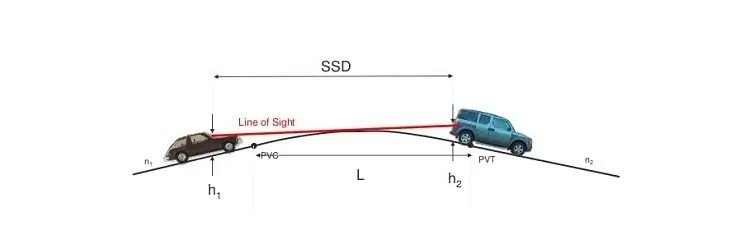The Summit curves are usually provided in the following cases.

2) – When the steeper upgrade is followed by a milder upgrade, &

3) – When the milder downgrade is followed by a steeper upgrade.

### b) – Sag Curve Or Valley Curve.

The vertical curve having its convexity in the downwards direction or when it is the concave upwards is known as a valley curve. It is also known as sag curve.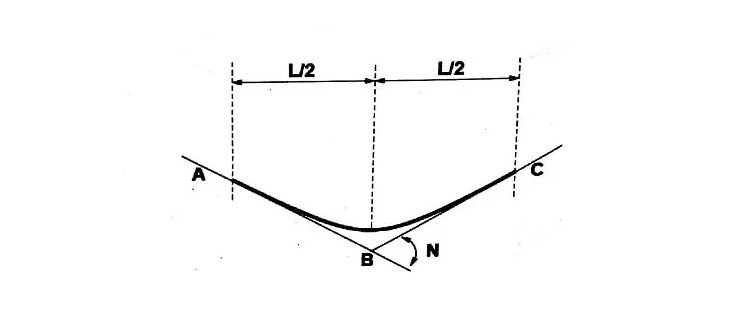The sag curve or a valley curve is usually formed in the following cases.

2) – When the steeper downgrade is followed by a milder upgrade, &

3) – When the milder upgrade is followed by a steeper upgrade.

### THANKS.